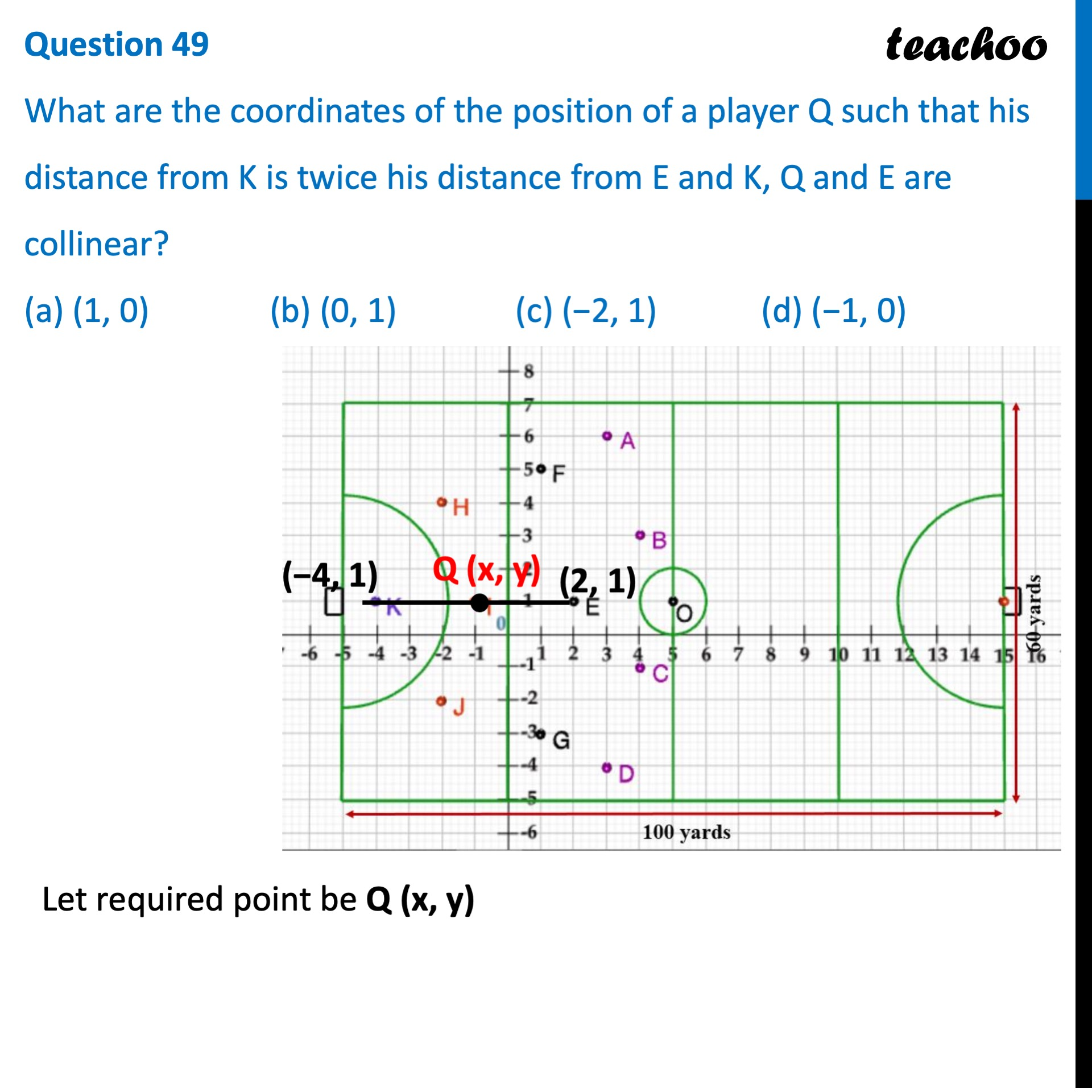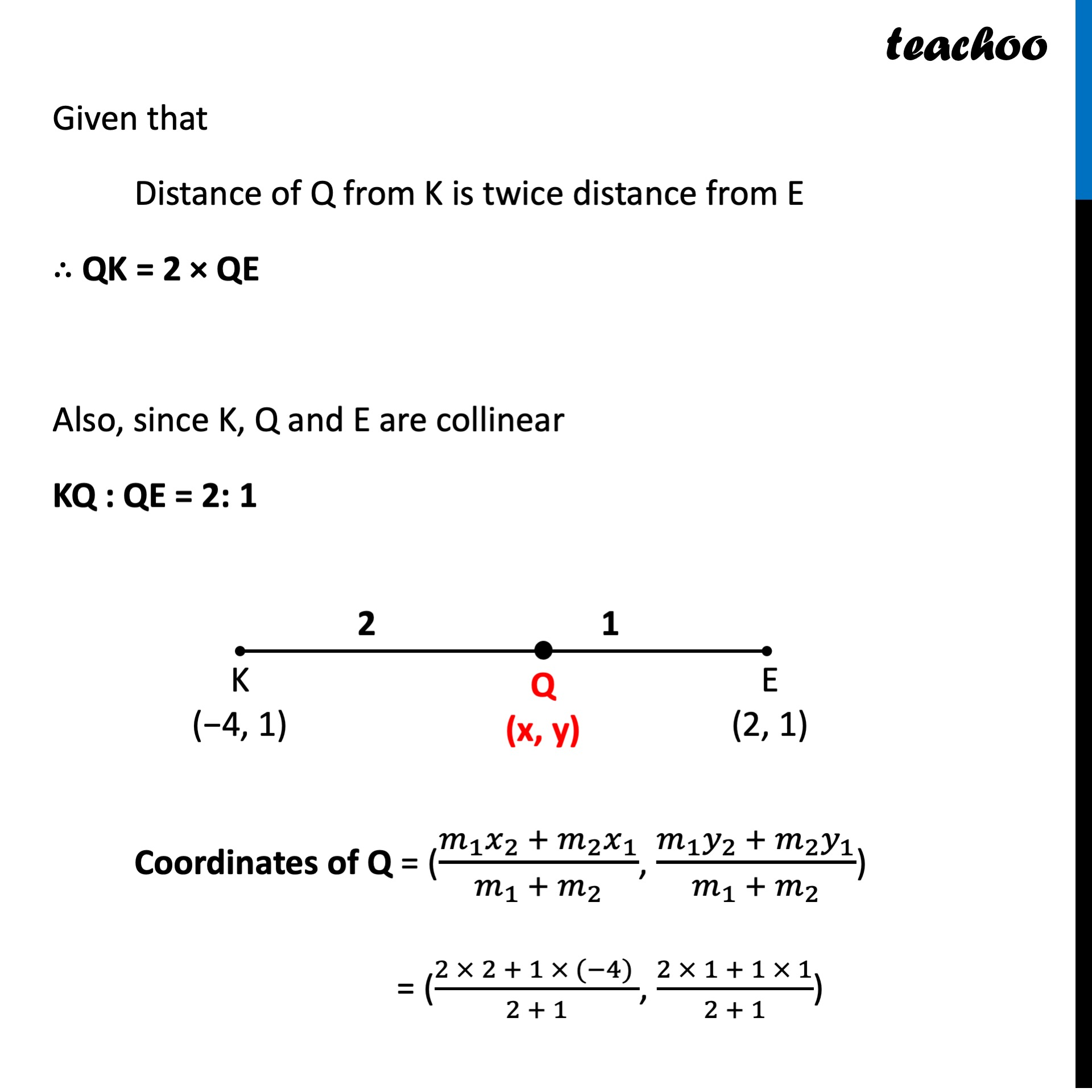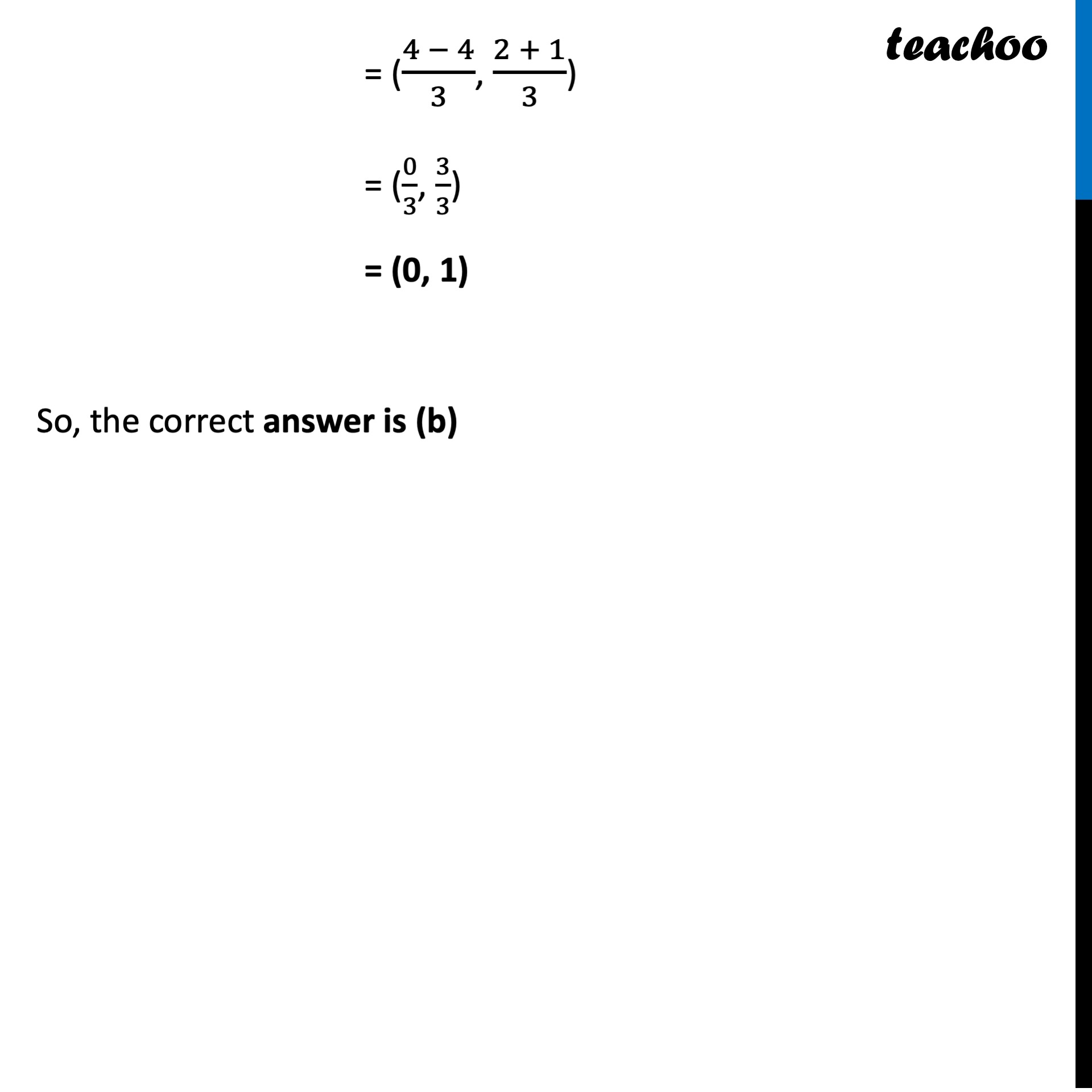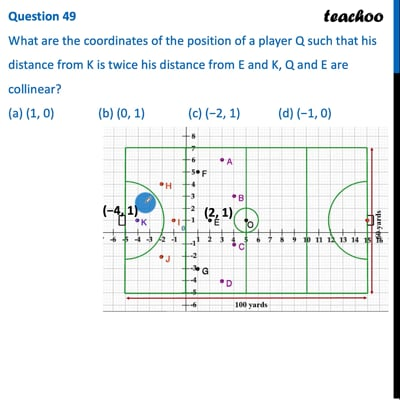CBSE Class 10 Sample Paper for 2022 Boards - Maths Standard [MCQ]

Class 10
Solutions of Sample Papers for Class 10 Boards

## (a) (1, 0)   (b) (0, 1)   (c) (−2, 1)   (d) (−1, 0)This video is only available for Teachoo black users

Introducing your new favourite teacher - Teachoo Black, at only ₹83 per month

### Transcript

Question 49 What are the coordinates of the position of a player Q such that his distance from K is twice his distance from E and K, Q and E are collinear? (a) (1, 0) (b) (0, 1) (c) (−2, 1) (d) (−1, 0) Let required point be Q (x, y) Given that Distance of Q from K is twice distance from E ∴ QK = 2 × QE Also, since K, Q and E are collinear KQ : QE = 2: 1 Coordinates of Q = ((𝑚_1 𝑥_2 + 𝑚_2 𝑥_1)/(𝑚_1 + 𝑚_2 ), (𝑚_1 𝑦_2 + 𝑚_2 𝑦_1)/(𝑚_1 + 𝑚_2 )) = ((2 × 2 + 1 × (−4) )/(2 + 1), (2 × 1 + 1 × 1)/(2 + 1)) = ((4 − 4)/3, (2 + 1)/3) = (0/3, 3/3) = (0, 1) So, the correct answer is (b)